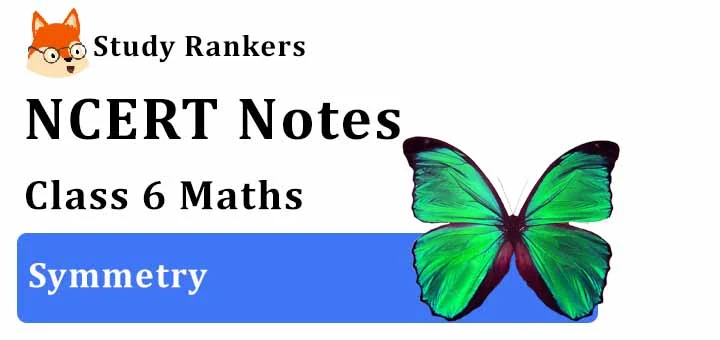# Chapter 13 Symmetry Class 6 Notes Maths

You will get Chapter 13 Symmetry Class 6 Notes Maths will ensure that remembering and retaining the syllabus more easy and efficient. You will understand the various factors through which one can improve their efficiency and eventually scores higher in the exam. NCERT Solutions for Class 6 Chapter 13 Maths that will be useful in the preparation of exams. Through these Class 6 Revision notes a student can boost their preparation and assessment of understood concepts.Line of symmetry

• Line of symmetry : A figure is said to be line of symmetry, if by folding the figure along a line, the left and right parts of it coincide exactly. This line is called the line (or axis) of symmetry of the figure.

• A figure may have no line of symmetry, one line of symmetry, two lines of symmetry, three lines of symmetry and so on.

Figures with no line of symmetry

• Scalene triangle does not have any line of symmetry. Because there is no line along which the Scalene triangle can be folded so that points of the Scalene triangle on one side of the line will coincide with points of the Scalene triangle on the other.

• Letter R has no line of symmetry.

Figures with one line of symmetry

• Letter A
• A Kite
• An isosceles triangle

Figures with two line of symmetry

• Letter H
• A Rhombus
• A Rectangle

Figures with more than two line of symmetry

• An equilateral triangle has three lines of symmetry.
• A square has four lines of symmetry.
• A circle has countless lines of symmetry.

Reflection and Symmetry

• The line symmetry is closely related to mirror reflection. When dealing with mirror reflection, we have to take into account the left ↔ right changes in orientation.

Applications in daily life

• Symmetry has plenty of applications in everyday life as in art, architecture, textile technology, design creations, geometrical reasoning, Kolams, Rangoli etc.